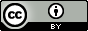3 minutes37

# Nernst Equation

Potential is a thermodynamic quantity reflecting the intrinsic driving force of a redox process, and it is directly related to the free energy change and equilibrium constant for the process. For redox processes taking place in electrochemical cells, the maximum (electrical) work done by the system is easily computed from the cell potential and the reaction stoichiometry and is equal to the free energy change for the process. The equilibrium constant for a redox reaction is logarithmically related to the reaction’s cell potential, with larger (more positive) potentials indicating reactions with greater driving force that equilibrate when the reaction has proceeded far towards completion (large value of K). Finally, the potential of a redox process varies with the composition of the reaction mixture, being related to the reactions standard potential and the value of its reaction quotient, Q, as described by the Nernst equation.

## 37.1 Potential, Free Energy, and Equilibrium

### Learning Objectives

By the end of this section, you will be able to:

• Use the Nernst equation to determine cell potentials under nonstandard conditions

### 37.1.1 Potentials at Nonstandard Conditions: The Nernst Equation

Most of the redox processes that interest science and society do not occur under standard state conditions, and so the potentials of these systems under nonstandard conditions are a property worthy of attention. Having established the relationship between potential and free energy change in this section, the previously discussed relation between free energy change and reaction mixture composition can be used for this purpose.

$\text{Δ}G=\text{Δ}G\text{°}+RT\phantom{\rule{0.2em}{0ex}}\text{ln}\phantom{\rule{0.2em}{0ex}}Q$

Notice the reaction quotient, Q, appears in this equation, making the free energy change dependent upon the composition of the reaction mixture. Substituting the equation relating free energy change to cell potential yields the Nernst equation:

$\text{−}nF{E}_{\text{cell}}=\text{−}nF{E}_{\text{cell}}^{°}+RT\phantom{\rule{0.2em}{0ex}}\text{ln}\phantom{\rule{0.2em}{0ex}}Q$
${E}_{\text{cell}}={E}_{\text{cell}}^{°}-\phantom{\rule{0.2em}{0ex}}\frac{RT}{nF}\phantom{\rule{0.4em}{0ex}}\text{ln}\phantom{\rule{0.3em}{0ex}}Q$

This equation describes how the potential of a redox system (such as a galvanic cell) varies from its standard state value, specifically, showing it to be a function of the number of electrons transferred, n, the temperature, T, and the reaction mixture composition as reflected in Q. A convenient form of the Nernst equation for most work is one in which values for the fundamental constants (R and F) and standard temperature (298) K), along with a factor converting from natural to base-10 logarithms, have been included:

${E}_{\text{cell}}={E}_{\text{cell}}^{°}-\phantom{\rule{0.2em}{0ex}}\frac{0.0592\text{V}}{n}\phantom{\rule{0.2em}{0ex}}\text{log}\phantom{\rule{0.2em}{0ex}}Q$

### EXAMPLE 37.1.2

#### Predicting Redox Spontaneity Under Nonstandard Conditions

Use the Nernst equation to predict the spontaneity of the redox reaction shown below.
$\text{Co}\left(s\right)+{\text{Fe}}^{2+}\left(aq,\phantom{\rule{0.2em}{0ex}}1.94\phantom{\rule{0.2em}{0ex}}M\right)\phantom{\rule{0.2em}{0ex}}⟶\phantom{\rule{0.2em}{0ex}}{\text{Co}}^{2+}\left(aq\text{, 0.15}\phantom{\rule{0.2em}{0ex}}M\right)+\text{Fe}\left(s\right)$

#### Solution

Collecting information from Appendix L and the problem,
$\begin{array}{}\\ \text{Anode (oxidation):}\hfill & \text{Co}\left(s\right)\phantom{\rule{0.2em}{0ex}}⟶\phantom{\rule{0.2em}{0ex}}{\text{Co}}^{2+}\left(aq\right)+{\text{2e}}^{\text{−}}\hfill & \hfill {E}_{{\text{Co}}^{2+}\text{/Co}}^{°}& =\hfill & \text{−0.28 V}\hfill \\ \text{Cathode (reduction):}\hfill & {\text{Fe}}^{2+}\left(aq\right)+{\text{2e}}^{\text{−}}\phantom{\rule{0.2em}{0ex}}⟶\phantom{\rule{0.2em}{0ex}}\text{Fe}\left(s\right)\hfill & \hfill {E}_{{\text{Fe}}^{2+}\text{/Fe}}^{°}& =\hfill & \text{−0.447 V}\hfill \\ & {E}_{\text{cell}}^{°}={E}_{\text{cathode}}^{°}-{E}_{\text{anode}}^{°}=\hfill & \hfill \text{−0.447 V}-\left(\text{−0.28 V}\right)& =\hfill & \text{−0.17 V}\hfill \end{array}$

Notice the negative value of the standard cell potential indicates the process is not spontaneous under standard conditions. Substitution of the Nernst equation terms for the nonstandard conditions yields:

$\begin{array}{ccc}\hfill Q& =\hfill & \frac{{\left[\text{Co}}^{2+}\right]}{{\left[\text{Fe}}^{2+}\right]}\phantom{\rule{0.2em}{0ex}}=\phantom{\rule{0.2em}{0ex}}\frac{0.15\phantom{\rule{0.2em}{0ex}}M}{1.94\phantom{\rule{0.2em}{0ex}}M}\phantom{\rule{0.2em}{0ex}}=0.077\hfill \\ \hfill {E}_{\text{cell}}& =\hfill & {E}_{\text{cell}}^{°}-\phantom{\rule{0.2em}{0ex}}\frac{0.0592 V}{n}\phantom{\rule{0.4em}{0ex}}\text{log}\phantom{\rule{0.2em}{0ex}}Q\hfill \\ \hfill {E}_{\text{cell}}& =\hfill & -0.1\text{7 V}-\phantom{\rule{0.2em}{0ex}}\frac{0.0592 V}{2}\phantom{\rule{0.4em}{0ex}}\text{log}\phantom{\rule{0.2em}{0ex}}0.077\hfill \\ \hfill {E}_{\text{cell}}& =\hfill & \text{−0.17 V}+0.033 V=-0.14 V\hfill \end{array}$

The cell potential remains negative (slightly) under the specified conditions, and so the reaction remains nonspontaneous.

For the cell schematic below, identify values for n and Q, and calculate the cell potential, Ecell.
$\text{Al}\left(s\right)│{\text{Al}}^{3+}\left(aq,\phantom{\rule{0.2em}{0ex}}0.15\phantom{\rule{0.2em}{0ex}}M\right)║{\text{Cu}}^{2+}\left(aq,\phantom{\rule{0.2em}{0ex}}0.025\phantom{\rule{0.2em}{0ex}}M\right)│\text{Cu}\left(s\right)$

n = 6; Q = 1440; Ecell = +1.97 V, spontaneous.

A concentration cell is constructed by connecting two nearly identical half-cells, each based on the same half-reaction and using the same electrode, varying only in the concentration of one redox species. The potential of a concentration cell, therefore, is determined only by the difference in concentration of the chosen redox species. The example problem below illustrates the use of the Nernst equation in calculations involving concentration cells.

### EXAMPLE 37.1.3

#### Concentration Cells

What is the cell potential of the concentration cell described by
$\text{Zn}\left(s\right)│{\text{Zn}}^{2+}\left(aq\text{, 0.10}\phantom{\rule{0.2em}{0ex}}M\right)║{\text{Zn}}^{2+}\left(aq\text{, 0.50}\phantom{\rule{0.2em}{0ex}}M\right)│\text{Zn}\left(s\right)$

#### Solution

From the information given:
$\begin{array}{}\\ \underset{¯}{\begin{array}{ccccccccc}\text{Anode:}\hfill & & & \text{Zn}\left(s\right)\phantom{\rule{0.2em}{0ex}}⟶\phantom{\rule{0.2em}{0ex}}{\text{Zn}}^{2+}\left(aq\text{, 0.10}\phantom{\rule{0.2em}{0ex}}M\right)+{\text{2e}}^{\text{−}}\hfill & & & \hfill {E}_{\text{anode}}^{°}& =\hfill & \text{−0.7618 V}\hfill \\ \text{Cathode:}\hfill & & & {\text{Zn}}^{2+}\left(aq\text{, 0.50}\phantom{\rule{0.2em}{0ex}}M\right)+{\text{2e}}^{\text{−}}\phantom{\rule{0.2em}{0ex}}⟶\phantom{\rule{0.2em}{0ex}}\text{Zn}\left(s\right)\hfill & & & \hfill {E}_{\text{cathode}}^{°}& =\hfill & \text{−0.7618 V}\hfill \end{array}}\hfill \\ \begin{array}{ccccccccc}\text{Overall:}\hfill & & & {\text{Zn}}^{2+}\left(aq\text{, 0.50}\phantom{\rule{0.2em}{0ex}}M\right)\phantom{\rule{0.2em}{0ex}}⟶\phantom{\rule{0.2em}{0ex}}{\text{Zn}}^{2+}\left(aq\text{, 0.10}\phantom{\rule{0.2em}{0ex}}M\right)\hfill & & & \hfill {E}_{\text{cell}}^{°}& =\hfill & \text{0.000 V}\hfill \end{array}\hfill \end{array}$

Substituting into the Nernst equation,

${E}_{\text{cell}}=\text{0.000 V}-\phantom{\rule{0.2em}{0ex}}\frac{\text{0.0592 V}}{2}\phantom{\rule{0.4em}{0ex}}\text{log}\phantom{\rule{0.4em}{0ex}}\frac{0.10}{0.50}\phantom{\rule{0.2em}{0ex}}=+0.021 V$

The positive value for cell potential indicates the overall cell reaction (see above) is spontaneous. This spontaneous reaction is one in which the zinc ion concentration in the cathode falls (it is reduced to elemental zinc) while that in the anode rises (it is produced by oxidation of the zinc anode). A greater driving force for zinc reduction is present in the cathode, where the zinc(II) ion concentration is greater (Ecathode > Eanode).

The concentration cell above was allowed to operate until the cell reaction reached equilibrium. What are the cell potential and the concentrations of zinc(II) in each half-cell for the cell now?

Ecell = 0.000 V; [Zn2+]cathode = [Zn2+]anode = 0.30 M

### Suggested Citation

General College Chemistry. https://edtechbooks.org/general_college_chemistry_2

Previous Version(s)

Flowers, P., et al. (2019). Chemistry: Atoms First 2e. https://openstax.org/details/books/chemistry-atoms-first-2e (16.4)CC BY: This work is released under a CC BY license, which means that you are free to do with it as you please as long as you properly attribute it.

### End-of-Chapter Survey

: How would you rate the overall quality of this chapter?
1. Very Low Quality
2. Low Quality
3. Moderate Quality
4. High Quality
5. Very High Quality
Comments will be automatically submitted when you navigate away from the page.